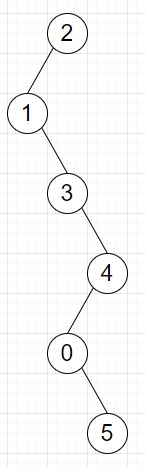# Program to convert linked list to zig-zag binary tree in Python

Suppose we have a singly linked list, we have to convert it to a binary tree path using following rules −

• Each subsequent node is the left child of the parent when its value is less, otherwise it will be the right child.

So, if the input is like [2,1,3,4,0,5], then the output will beTo solve this, we will follow these steps −

• Define a function solve() . This will take node
• if node is null, then
• return null
• root := create a tree node with value same as value of node
• if next of node is not null, then
• if value of next of node < value of node, then
• left of root := solve(next of node)
• otherwise,
• right of root := solve(next of node)
• return root

Let us see the following implementation to get better understanding −

## Example

Live Demo

class ListNode:
def __init__(self, data, next = None):
self.val = data
self.next = next
def make_list(elements):
for element in elements[1:]:
while ptr.next:
ptr = ptr.next
ptr.next = ListNode(element)
class TreeNode:
def __init__(self, data, left = None, right = None):
self.data = data
self.left = left
self.right = right
def print_tree(root):
if root is not None: print_tree(root.left)
print(root.data, end = ', ') print_tree(root.right)
class Solution:
def solve(self, node):
if not node:
return None
root = TreeNode(node.val)
if node.next:
if node.next.val < node.val:
root.left = self.solve(node.next)
else:
root.right = self.solve(node.next)
return root
ob = Solution()
L = make_list([2,1,3,4,0,5])
print_tree(ob.solve(L))

## Input

[2,1,3,4,0,5]

## Output

1, 3, 0, 5, 4, 2,

Updated on: 19-Nov-2020

116 Views